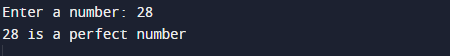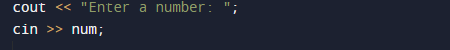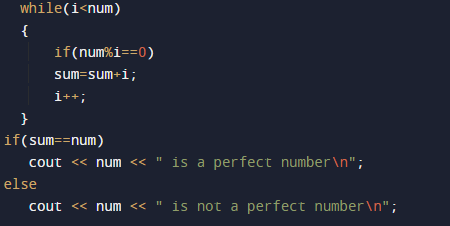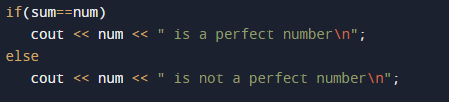# C++ Program to Find Perfect Number

In this tutorial you will learn about the C++ Program to Find Perfect Number and its application with practical example.

In this tutorial, we will learn to create a c++ program that will find what is A Prefect Number using c++ programming.

## Prerequisites

Before starting with this tutorial we assume that you are best aware of the following C programming topics:

•  Operators.
• Looping statements.
• C++ While Loop.
• Basic input/output.

## What Is Perfect Number?

Perfect number is a positive integer number,that equals to sum of its positive divisors not include the number itself is equal to that number.

## Example

Divisor of  28 are 1 + 2 + 4 + 7 + 14. and sum of these  are (1+2+4+7+14=28). So we can say that 28 is a perfect number in which we will not induced 28 i.e exclude 28 .So number is  called perfect when divisor of number added and that create the number itself called Perfect number.

## C++ Program to Find Perfect Number.

So here we are going to create a program in which we take a value from user and we will find the given number is perfect or not? By method using adding all divisors of a number.

## OutputIn the above program, we have first declared and initialized a set variables required in the program.

• num  =  holding given number
• i       = for iteration of a loop.

Explanation of program, First we take the integer value in ‘num’ variable. So the condition is that A Perfect number is a number which is equal to sum of its divisor.Example, 6’s divisor  are 1, 2 and 3. and there sum is (1+2+3=6) ,the divisors of  6. So the number 6 is called as perfect number.

From the above image of a perfect number in sum,Suppose  user entered value is: no = 6
Step by step explanation for loop is here.In 1st Iteration…
Number is num= 6, sum = 0 and loop start at i = 1.
If (no % i == 0)  // if( 6%1== 0 ) true.
here number is complete divisible So,
sum = sum + i  // sum= 0 + 1 =1.

Now,Second Iteration..
Values of  sum and i has altered as sum = 1 and i = 2.

If (no % i == 0) // if( 6 %2 ==0 ) true.
sum = sum + i // sum = 1 + 2 = 3.

Third Iteration.
Again the values of both sum and i has altered as sum = 3 and i = 3

If (6 % 3 == 0)  // if( 6%3==0) true.
sum = sum + i //sum = 3 + 3 = 6.

Next fourth and fifth iterations ,condition inside the if will fail
6 % 4 == 0 (FALSE)
6 % 5 == 0 (FALSE)

In next iteration, the value of i becomes 6, So (6 < 6) false . So, the compiler will terminate the for loop.
Then, we check whether the value of sum  is exactly equal to the no or Not.If it is TRUE, the below cout<<” is a perfect number”  statement will execute. else cout<<” is not a perfect number” statement will execute.

In this tutorial we have learn about the C++ Program to Find Perfect Number and its application with practical example. I hope you will like this tutorial.